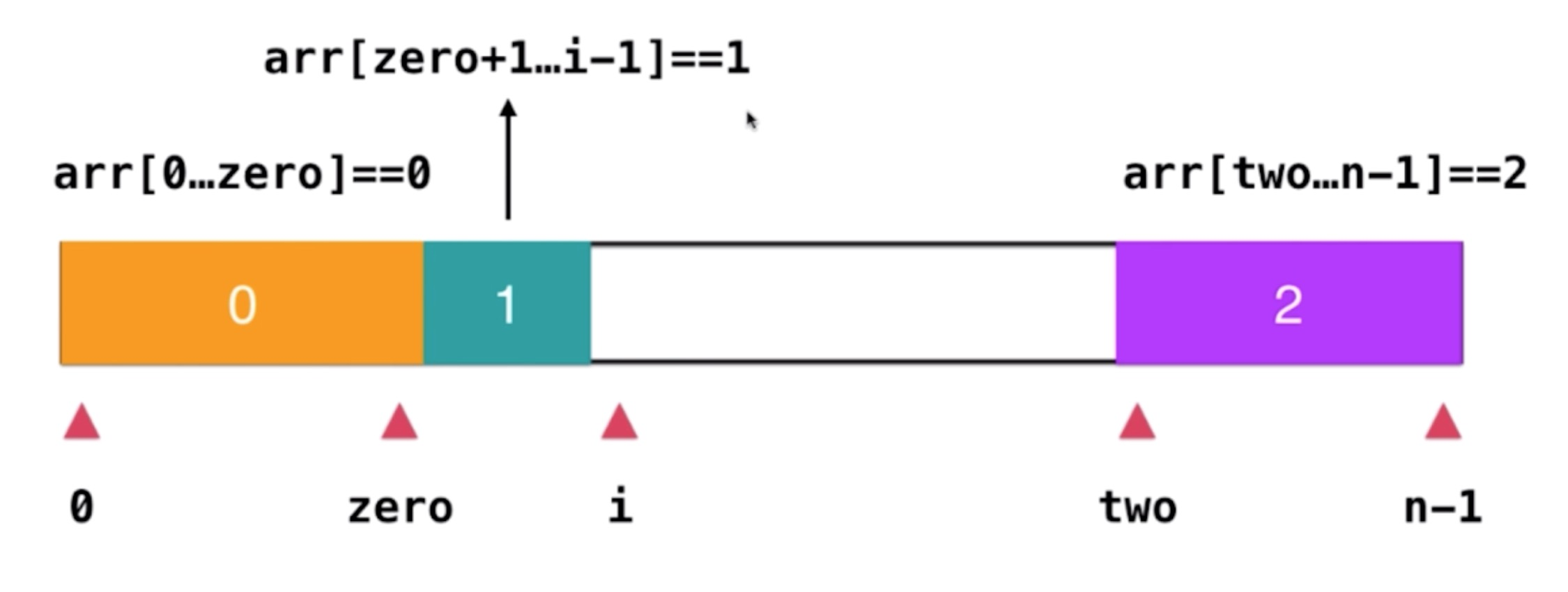Time Flying### 题目

``````输入: [2,0,2,1,1,0]

### 解题

``````输入
[2,0,2,1,1,0]

[0,1,2,1,1,0]

[0,0,1,1,2,2]``````
``````/**
* @param {number[]} nums
* @return {void} Do not return anything, modify nums in-place instead.
*/
var sortColors = function (nums) {
let countObj = {}
for (let i = 0; i < nums.length; i++) {
const key = nums[i]
if (typeof(countObj[`\${key}`]) === 'number') {
countObj[`\${key}`] = countObj[`\${key}`] + 1
} else {
countObj[`\${key}`] = 1
}
}

const count0 = countObj || 0
const count1 = countObj || 0
const count2 = countObj || 0
for (let i = 0; i < count0 + count1 + count2; i++) {
if (i < count0) {
nums[i] = 0
} else if (i >=count0 && i < count0 + count1) {
nums[i] = 1
} else if (i >= count1 && i < count0 + count1 + count2) {
nums[i] = 2
}
}
}````````````/**
* @param {number[]} nums
* @return {void} Do not return anything, modify nums in-place instead.
*/
var sortColors = function (nums) {
/**
* nums[0, zero] = 0
* nums[two, nums.length - 1] = 2
*/
let [zero, two] = [0, nums.length - 1]
for (let i = 0; i <= two; i++) {
if (nums[i] === 0) {
[nums[i], nums[zero]] = [nums[zero], nums[i]]
zero++
}

if (nums[i] === 2) {
[nums[i], nums[two]] = [nums[two], nums[i]]
i--
two--
}
}
}``````

88、215